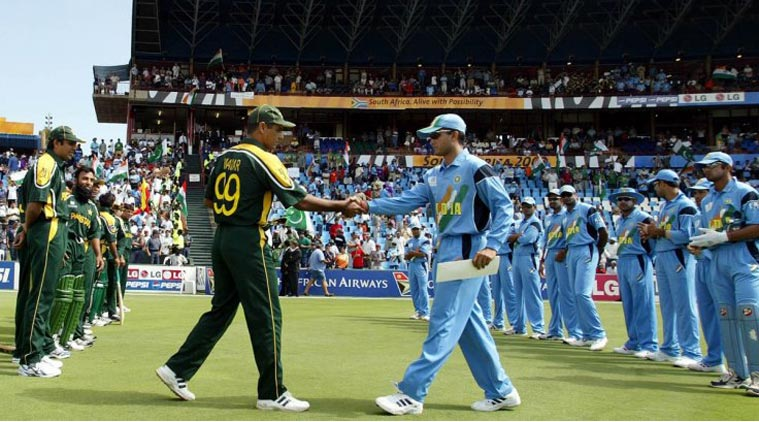# India vs Pak

Algebra Level 4India after finishing their batting , challenged a target of $300$ runs in $50$ overs against Pakistan in cricket World Cup $2015$.Now , to win the match , Pakistan had to do $301$ runs in $50$ overs. However , Pakistan did not manage to catch upto $301$ runs but all their players were out when they made $224$ runs. At that time , $3$ overs were still remaining. Let $R_1$ be the required run-rate for Pakistan to win the match by scoring the runs to win the match runs in 3 overs, $R_2$ was their current run-rate .

If $R_1 - R_2$ can be expressed as $\frac{a}{b}$ where , $a , b$ are co-prime positive integers , Find $a + b$

$Details$ $And$ $Assumptions:$ $RunRate = \frac{Number,of,runs,made}{Overs,consumed}$

Also try My 200 followers problem!

×

Problem Loading...

Note Loading...

Set Loading...